## Design MPC Controller for Identified Plant Model

You can define the internal plant model of your model predictive controller using a linear model identified using System Identification Toolbox™ software. You can identify the plant model and design the MPC controller interactively using apps or programmatically at the command line. For more information on identifying plant models, see Identify Plant from Data.

The System Identification app is not supported in MATLAB® Online™.

### Design Controller for Identified Plant Using Apps

This example shows how to interactively design a model predictive controller using an identified plant model. First, estimate the plant model from data using the System Identification app. Then design an MPC controller by importing the identified plant into MPC Designer.

Load the input and output data for identification.

` load(fullfile(matlabroot,'examples','mpc','plantIO'))`

This command imports the plant input signal, `u`, output signal, `y`, and sample time, `Ts`, to the MATLAB workspace.

Open the System Identification app.

`systemIdentification`

In the System Identification app, under Import data, select `Time domain data`.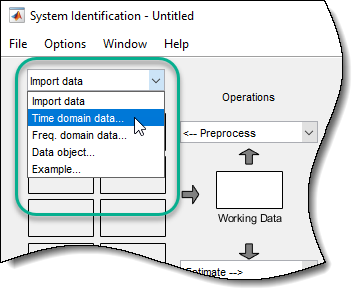In the Import Data dialog box, specify the Input, Output, and Sample time using the data from the MATLAB workspace.

Also, specify the Data name as `ioData` and Starting time as `0`.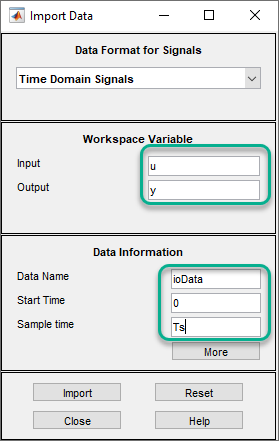Click Import. The app imports the data, creates an `iddata` object with the specified name and signal properties, and adds this object to the Data Views area.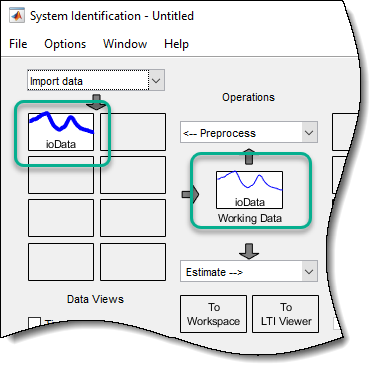Preprocess Data

Typically, you must preprocess identification I/O data before estimating a model. For this example, remove the offsets from the input and output signals by detrending the data. In the System Identification app, under Preprocess, select `Remove trends`.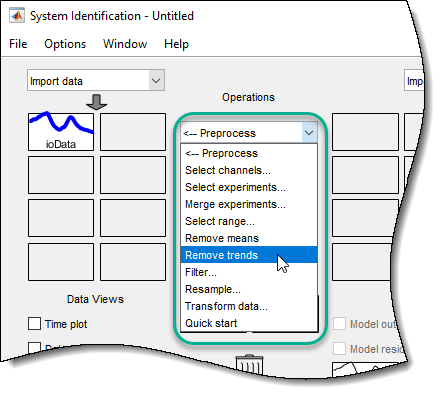The app creates a data object, `ioDatad`, using the preprocessed data, and adds this object to the Data Views area.

For more information on preprocessing identification data, see Preprocess Data (System Identification Toolbox).

Estimate Linear Model

To use the detrended data, `ioDatad`, for model estimation, first drag the corresponding data object from the area to Working Data.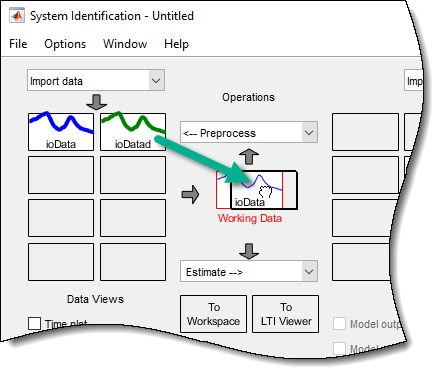To estimate a state-space model, under Estimate, select `State Space Models`.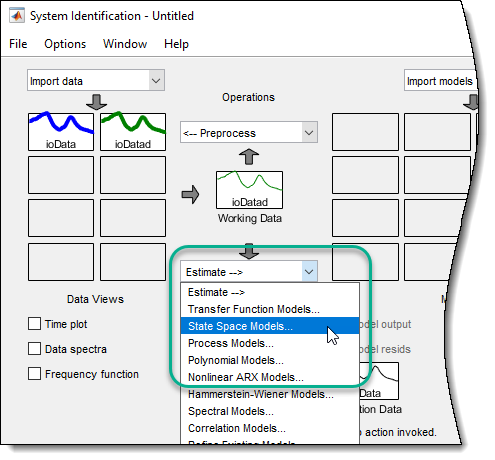In the State Space Models dialog box, specify the properties of the estimated model and the estimation options. For this example, estimate a second-order, discrete-time model, leaving the other estimation options at their default values.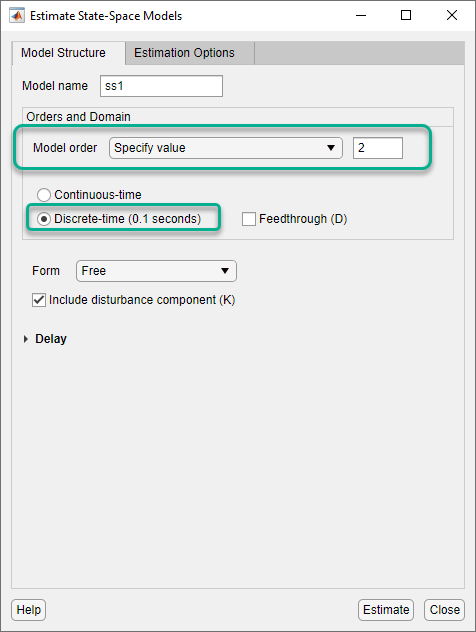For more information on estimating state-space models, see State-Space Models (System Identification Toolbox).

Click . The app estimates a state-space model, `ss1`, and adds the model to the Model Views area.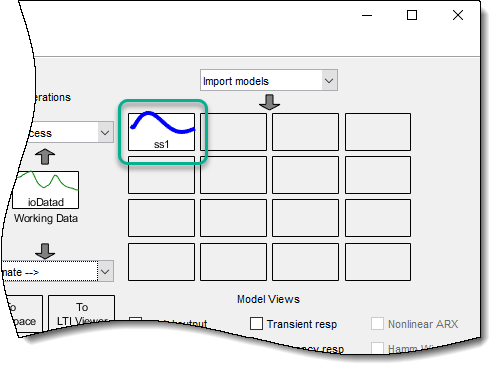The estimated model has one measured input and one unmeasured noise component.

Import Identified Plant to MPC Designer

To use `ss1` for MPC control design, first export the model to the MATLAB workspace.

Drag `ss1` from the Model Views area to To Workspace.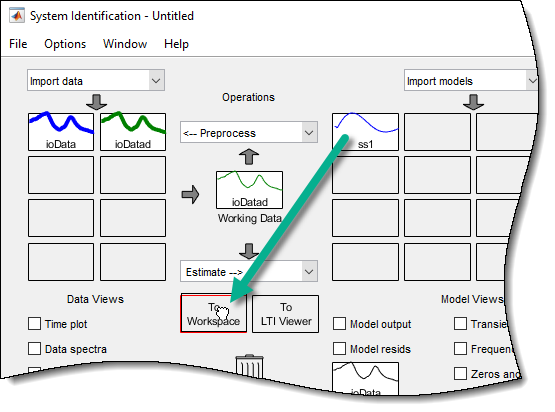Open MPC Designer. At the MATLAB command line, type:

`mpcDesigner`

To import the identified model, in MPC Designer, click MPC Structure. In the Define MPC Structure By Importing dialog box, select `ss1` from the table.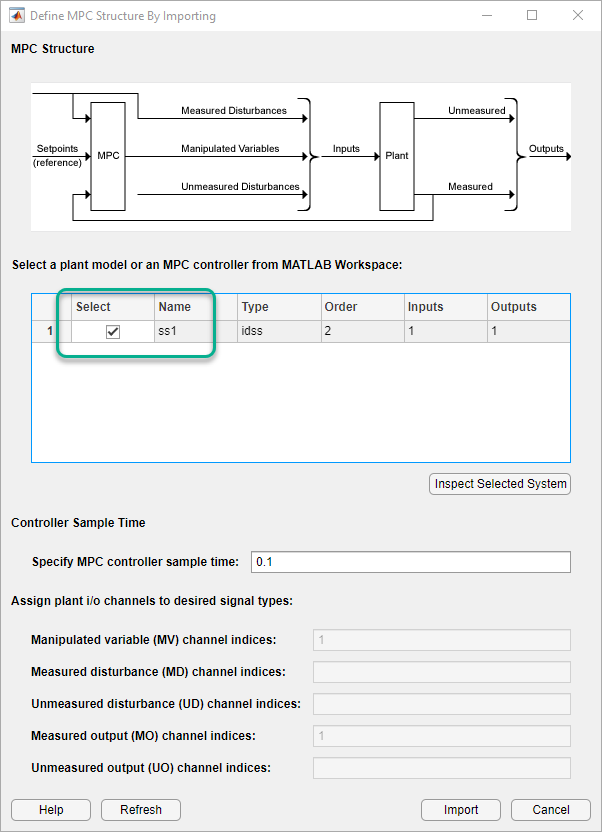Click .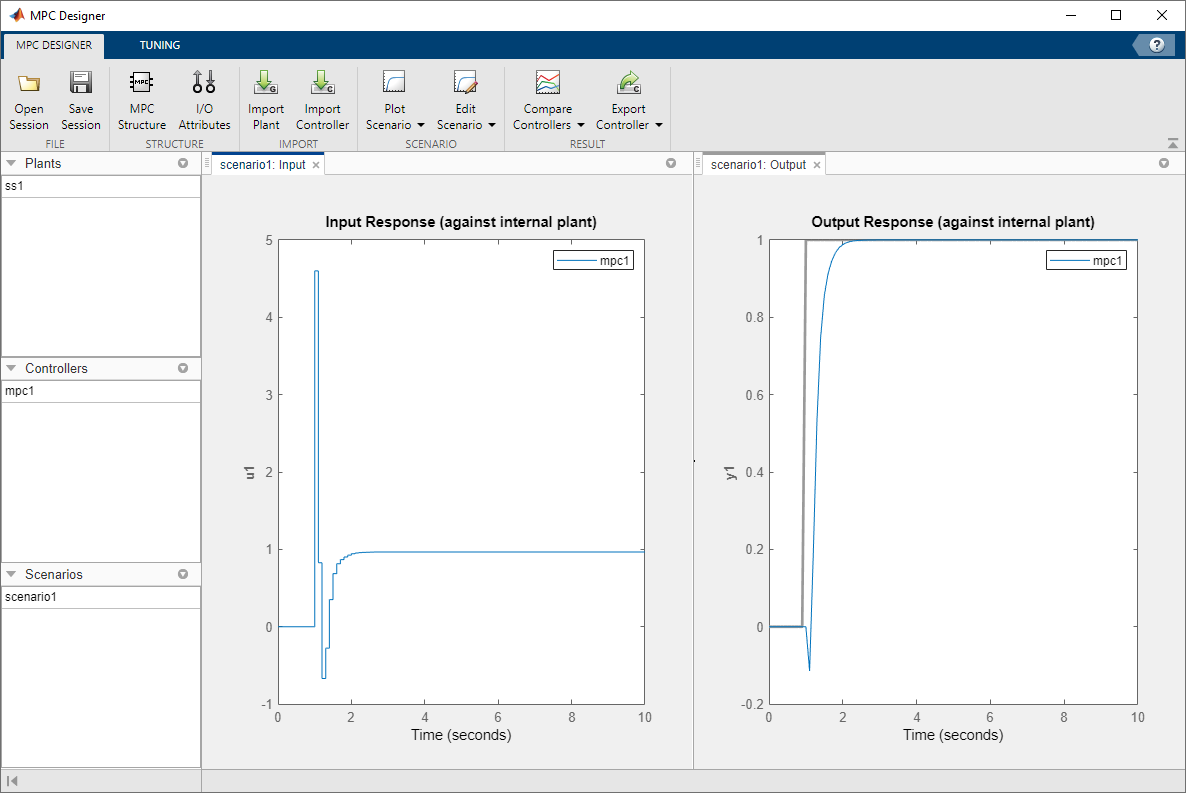Tip

You can also import the identified model when opening MPC Designer.

`mpcDesigner(ss1)`

The app converts the identified plant to a discrete-time, state-space model, if necessary, and creates a default MPC controller, `mpc1`, in which the:

• Measured input of the identified plant is a manipulated variable.

• Output of the identified plant is a measured output.

By default, the MPC controller discards the unmeasured noise component from your identified model. To configure noise channels as unmeasured disturbances, you must first create an augmented state-space model from your identified model. For more information, see Configure Noise Channels as Unmeasured Disturbances.

Note

You can also import an identified linear model into an existing MPC Designer session. In MPC Designer, click Import Plant. In the Import Plant Model dialog box, select an identified model from the table.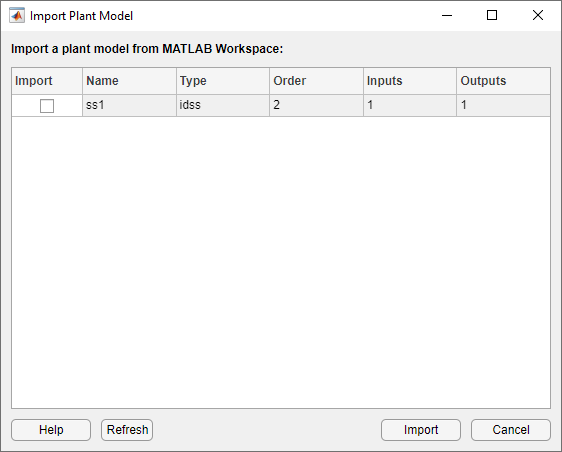Only identified models with an I/O configuration that is compatible with the current MPC structure are displayed in the Import Plant Model dialog box. If the current MPC structure includes unmeasured disturbances, any noise channels from the identified model are converted to unmeasured disturbances. Otherwise, the noise channels are discarded.

Specify I/O Attributes

To improve controller performance and simplify controller tuning, specify the following attributes for each input and output signal:

• Scale Factor — Scale each signal by a factor that approximates its span, which is the difference between its maximum and minimum values. Scaling simplifies controller weight tuning and improves the numerical conditioning of the controller. For more information, see Specify Scale Factors.

• Nominal Value — Apply an offset to each signal that corresponds to the nominal operating conditions under which you collected the identification data; that is the offsets removed by detrending the data. Specifying nominal values places the controller at the same operating point as the plant, which is important when the plant is a nonlinear system.

In MPC Designer, on the MPC Designer tab, click I/O Attributes.

In the Input and Output Channel Specifications dialog box, specify the Nominal Value and Scale Factor for the input and output signals.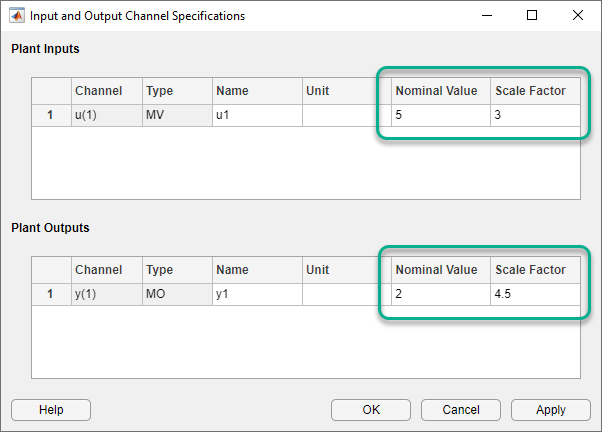Click .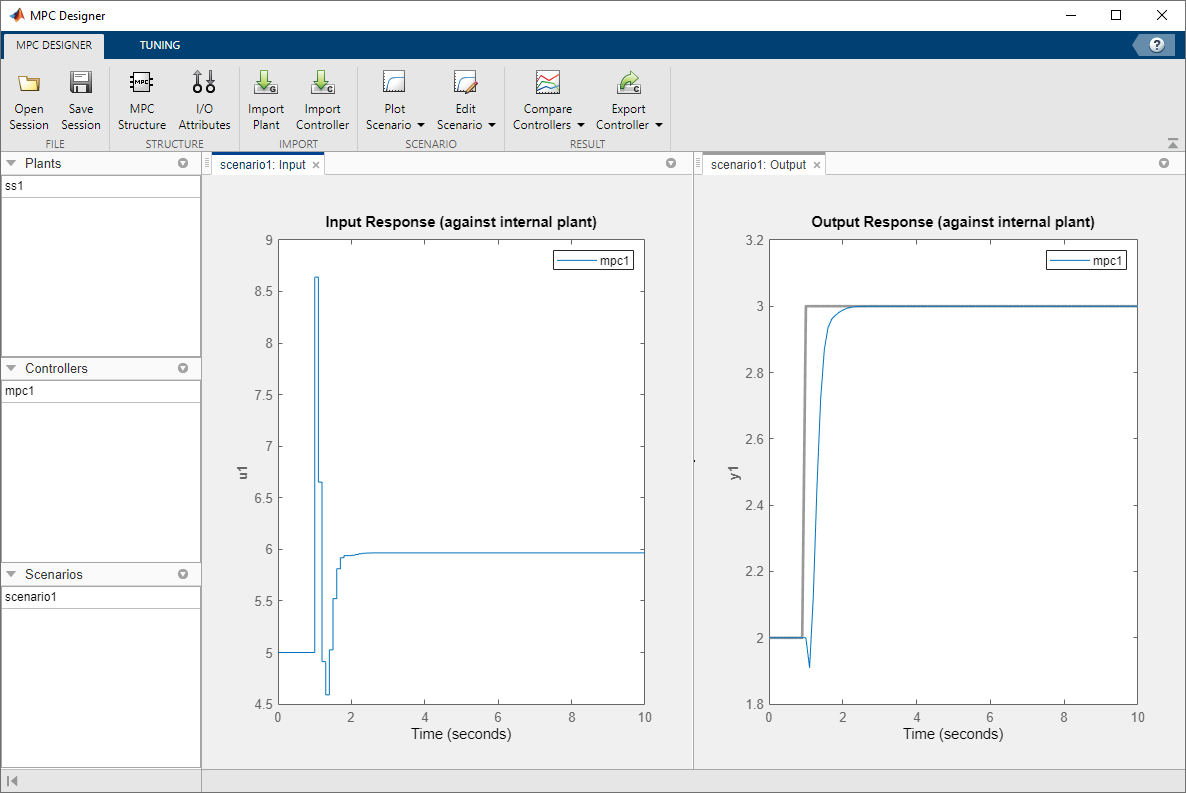The default controller tracks the output reference value well, however the initial controller response is aggressive.

Tip

You can specify the Nominal Value or Scale Factor using expressions such as `mean(u)` or `max(y) - min(y)` respectively, where `u` and `y` are the I/O signals from the MATLAB workspace.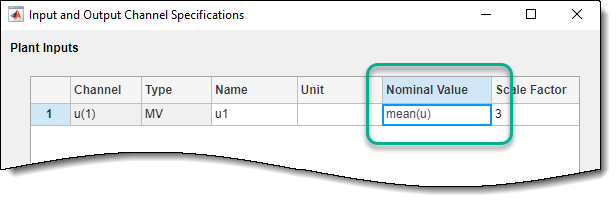Configure Simulation Scenario

In MPC Designer, on the Tuning tab, click Edit Scenario > scenario1.

In the Simulation Scenario dialog box, specify a Simulation duration of `5` seconds.

In the Reference Signals section, keep the default step signal.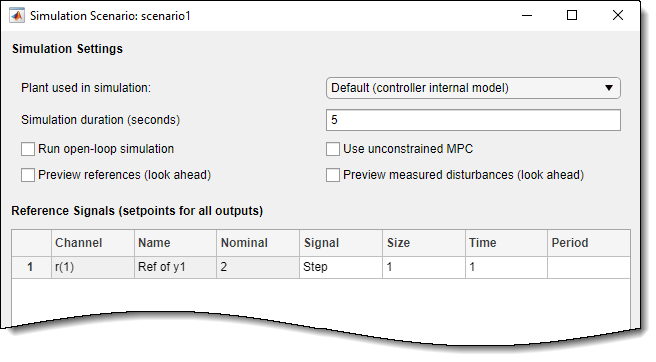Click

Tune Controller

Before tuning your controller, it is good practice to specify the controller sample time, prediction horizon, and control horizon. Since you identified a discrete-time plant model, the controller automatically derives its sample time from the identified model. For this example, use the default prediction and control horizons. For more information, see Choose Sample Time and Horizons.

To make the controller less aggressive, on the Tuning tab, drag the Closed-Loop Performance slider to the left. Doing so increases the cost function weight on the manipulated variable rate of change, and decreases the weight on the output variable.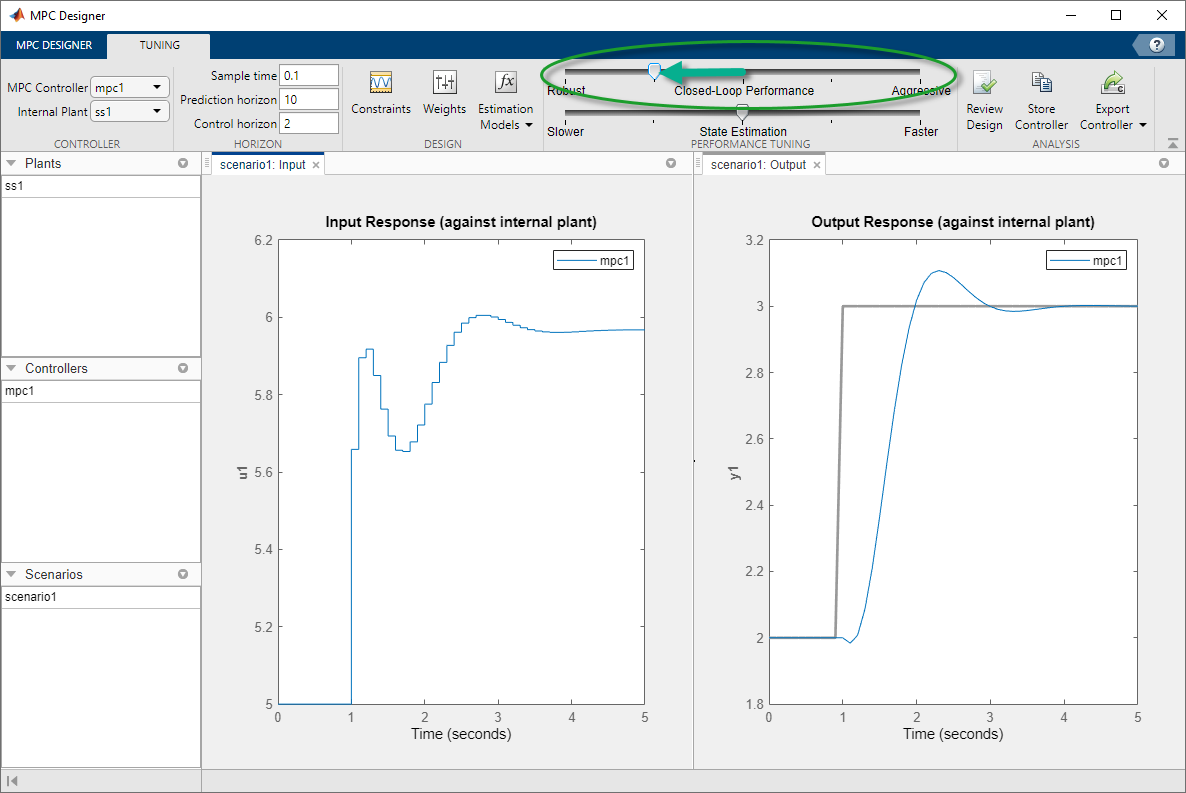The input response is now more conservative. The trade-offs are an increased overshoot and longer settling time.

Note

If your plant has known physical or safety constraints that limit the output range, input range, or input signal rate of change, you can specify these constraints in the MPC controller. If so, define the constraints before tuning your controller weights. For more information, see Specify Constraints.

### Design Controller for Identified Plant at the Command Line

This example shows how to design a model predictive controller at the command line using an identified plant model.

`load plantIO`

This command imports the plant input signal, `u`, plant output signal, `y`, and sample time, `Ts`, to the MATLAB® workspace.

Create an `iddata` object from the input and output data.

`mydata = iddata(y,u,Ts);`

Preprocess the I/O data by removing offsets (mean values) from the input and output signals.

`mydatad = detrend(mydata);`

You can also remove offsets by creating an `ssestOptions` object and specifying the `InputOffset` and `OutputOffset` options.

Estimate a second order, linear state-space model using the I/O data. Estimate a discrete-time model by specifying the sample time as `Ts`.

`ss1 = ssest(mydatad,2,'Ts',Ts);`

The estimated model has one measured input and one unmeasured noise component.

Create a default model predictive controller for the identified model, `ss1`.

`mpcObj = mpc(ss1);`
```-->Converting linear model from System Identification Toolbox to state-space. -->The "PredictionHorizon" property of "mpc" object is empty. Trying PredictionHorizon = 10. -->The "ControlHorizon" property of the "mpc" object is empty. Assuming 2. -->The "Weights.ManipulatedVariables" property of "mpc" object is empty. Assuming default 0.00000. -->The "Weights.ManipulatedVariablesRate" property of "mpc" object is empty. Assuming default 0.10000. -->The "Weights.OutputVariables" property of "mpc" object is empty. Assuming default 1.00000. ```

By default the controller discards the unmeasured noise component from your identified model.

To simplify the tuning process, specify input and output signal scaling factors.

```mpcObj.MV(1).ScaleFactor = max(u) - min(u); mpcObj.OV(1).ScaleFactor = max(y) - min(y);```

Specify the nominal values for the input and output signals. Use the offsets that you previously removed from the I/O data.

```nominalInput = mean(u); nominalOutput = mean(y); mpcObj.Model.Nominal.u = nominalInput; mpcObj.Model.Nominal.y = nominalOutput;```

Configure the simulation reference signal. Specify a reference signal with a five-second duration and a unit step at a time of one second. The initial value of the reference signal is the nominal value of the output signal.

```outputRef = [nominalOutput*ones(1/Ts,1); (nominalOutput+1)*ones(4/Ts+1,1)];```

Before tuning the controller, simulate the initial controller performance.

`sim(mpcObj,[],outputRef)`
```-->Assuming output disturbance added to measured output channel #1 is integrated white noise. -->The "Model.Noise" property of the "mpc" object is empty. Assuming white noise on each measured output channel. ```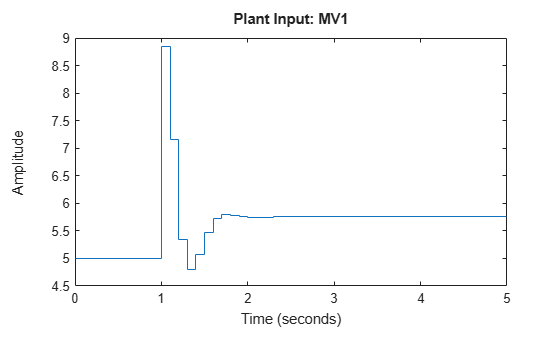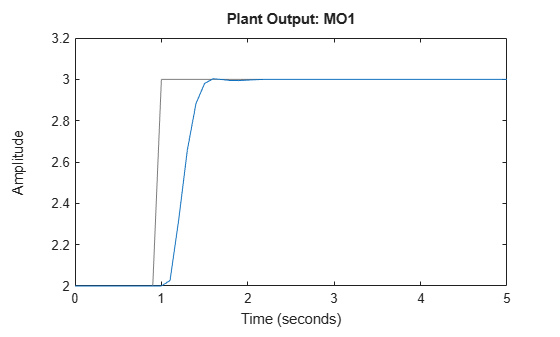The default controller tracks the output reference value well, however the initial controller response is aggressive.

To make the controller less aggressive, simultaneously increase the tuning weight for the manipulated variable rate of change and decrease the tuning weight for the output variable.

```beta = 0.37; mpcObj.Weights.MVRate = mpcObj.Weights.MVRate/beta; mpcObj.Weights.OV = mpcObj.Weights.OV*beta;```

Simulate the tuned controller response

`sim(mpcObj,[],outputRef)`
```-->Assuming output disturbance added to measured output channel #1 is integrated white noise. -->The "Model.Noise" property of the "mpc" object is empty. Assuming white noise on each measured output channel. ```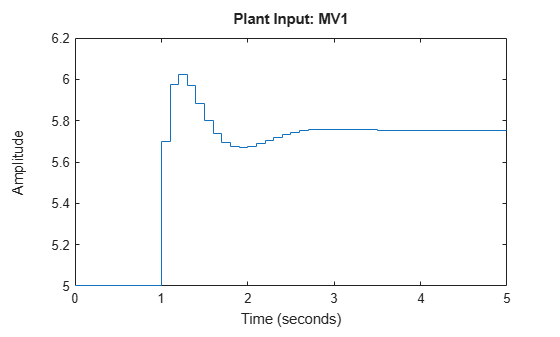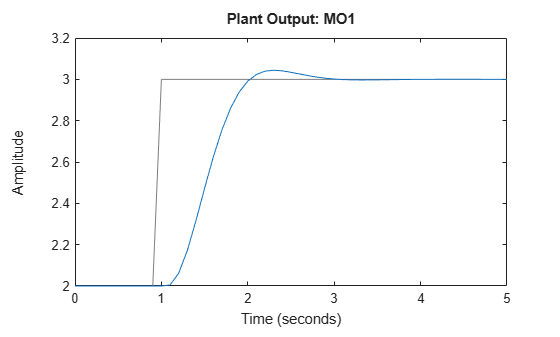The input response is now more conservative. The trade-offs are an increased overshoot and longer settling time.

### Configure Noise Channels as Unmeasured Disturbances

When you create an MPC controller using an identified model, the software discards any noise channels from the model by default. You can configure the noise channels as unmeasured disturbances by augmenting the identified model.

#### Augment Identified Model with Noise Channels

To convert noise channels to unmeasured disturbances, first convert the identified model, `ss1`, to a state-space model using the `'augmented'` option. At the MATLAB command line, type:

`ss2 = ss(ss1,'augmented');`

This option creates a state-space model, `ss2`, with the following input groups:

• `Measured` — The input channels from the identified model.

• `Noise` — The noise channels from the identified model. The number of noise channels matches the number of outputs channels.

Note

The System Identification Toolbox software assumes that the inputs to the `Noise` channels are unit-variance Gaussian noise. Therefore, the augmented model encapsulates any noise dynamics from the identified model, such as integration at the disturbance source.

You can then create an MPC controller using the augmented state-space model.

`mpcObj = mpc(ss2);`

The software configures the `Measured` inputs as manipulated variables and the `Noise` inputs as unmeasured disturbances.

You can also import the augmented model into MPC Designer.

`mpcDesigner(ss2)`

To view the MPC signal configuration, in MPC Designer, on the MPC Designer tab, click MPC Structure.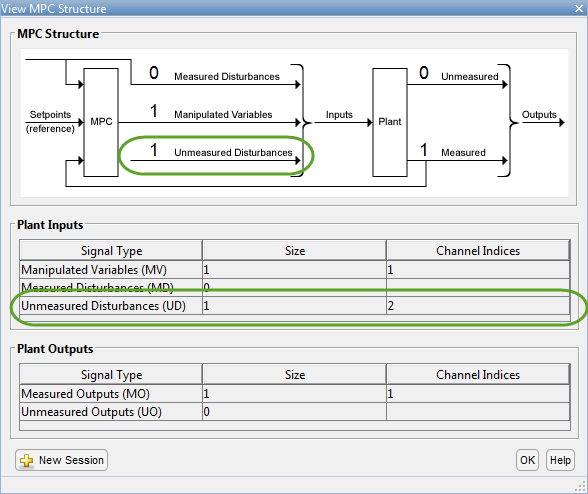The View MPC Structure dialog box shows the noise channels as unmeasured disturbances.

#### Configure Input Disturbance Model

When you convert an identified model to an augmented state-space model, the System Identification Toolbox software assumes that noise sources are unit-variance Gaussian noise. However, by default, MPC controllers model unmeasured input disturbances as integrated Gaussian noise. When designing your controller, you can:

• Remove the integrators from the input disturbance model, which simplifies the controller. Use this option if the experimental identification data was collected under conditions that closely match the expected plant operating conditions. In this case, the augmented state-space model encapsulates any noise dynamics from the identified system.

• Keep the default integrated white noise input disturbance model, which leads to more aggressive disturbance rejection. Use this option if the experimental identification data was collected under controlled conditions that may not match the expected plant operating conditions. In this case, the controller compensates for noise dynamics that the augmented model does not encapsulate.

Note

When using MPC Designer, you can tune your controller disturbance rejection properties by adjusting the State Estimation slider. For more information, see Disturbance Rejection Tuning.

To remove an integrator from an input disturbance model channel, configure that channel as a static unit gain. For example, to remove the integrators from all input disturbance model channels, set the input disturbance model to a static gain identity matrix. At the MATLAB command line, type:

`setindist(mpcObj,ss(eye(Nd)));`

where `Nd` is the number of unmeasured disturbances.

To set the disturbance model for an unmeasured disturbance channel to a static unit gain using MPC Designer:

1. On the Tuning tab, select Estimation Models > Input Disturbance Model.

2. In the Input Disturbance Model dialog box, in the Update the model drop-down list, select ```specifying a custom model channel by channel```.

3. In the Specifications table, in the Disturbance drop-down list, select ```White Noise```.

4. Specify a Magnitude of `1`.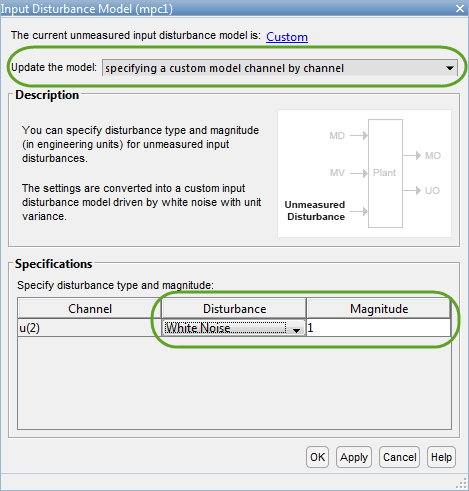5. Repeat steps 3 and 4 for each unmeasured disturbance.

6. To apply the changes and update the input disturbance model, click or .

#### Configure Simulation Scenario

You can simulate your MPC controller using unit-variance Gaussian noise unmeasured disturbance signals, as assumed by the System Identification Toolbox software. This scenario emulates the experimental conditions under which the data was collected for identification.

To configure unmeasured disturbance signals, create an MPC simulation option set for your controller using `mpcsimopt`. At the MATLAB command line, type:

`opt = mpcsimopt(mpcObj);`

Configure the `UnmeasuredDisturbance` option using `randn`.

`opt.UnmeasuredDisturbance = randn(T,Nd);`

where `T` is the number of simulation steps and `Nd` is the number of unmeasured disturbances.

Simulate the controller using this option set and an output reference signal, `outputRef`.

`y = sim(mpcObj,T,outputRef,opt);`

To configure your simulation in MPC Designer:

1. On the Tuning tab, under Edit Scenario select the simulation scenario you want to edit.

2. In the Simulation Scenario dialog box, in the Unmeasured Disturbances section, under Signal, select `Gaussian`.

3. Specify a Size of `1`, which corresponds to a unit variance.

4. To apply the disturbance from the start of the simulation, specify a Time of `0`.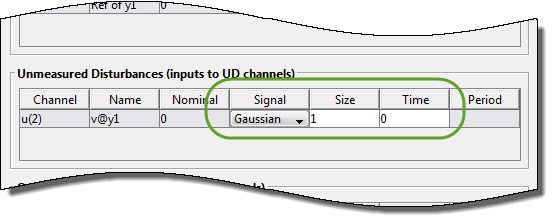5. Repeat steps 2–4 for each unmeasured disturbance channel.

6. To apply the changes and update the MPC Designer response plots, click or .